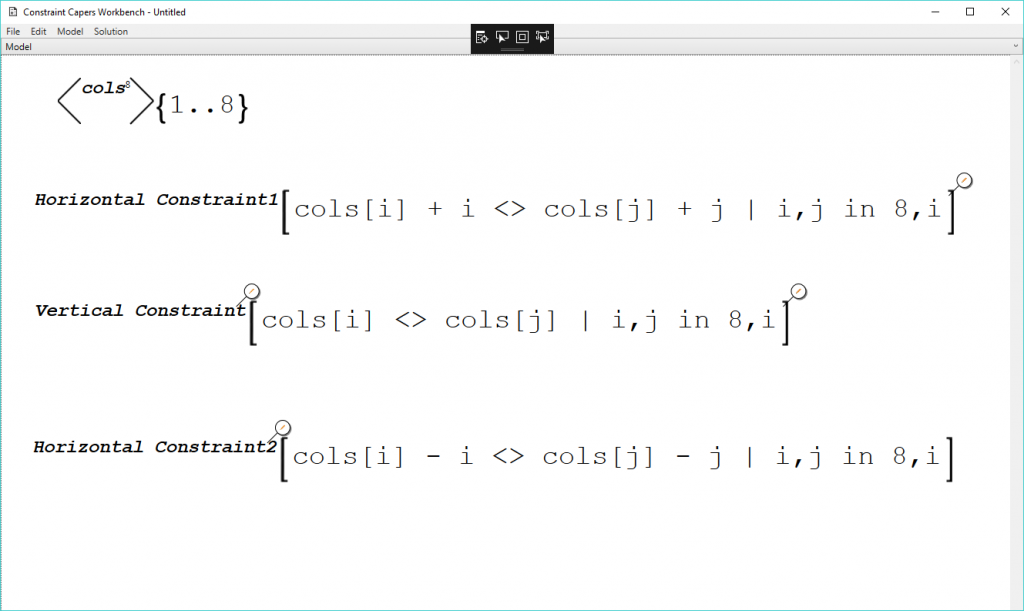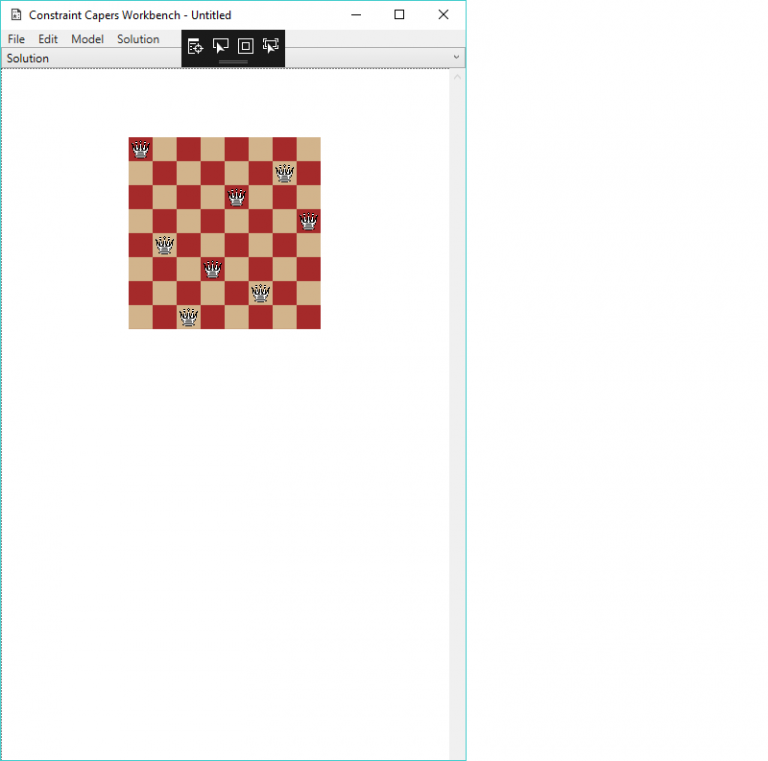It has taken quite a while, but version 0.3 of Constraint Capers Workbench is coming together pretty well.I decided that for this release I needed to start solving actual constraint problems. Well, one specific problem to be more precise, the classic problem, n-queens.

## Constraint Repeaters

Constraint repeaters provide a mechanism to expand a single constraint into many statements.

The n-queens model has the following constraint:

`cols[i] <> cols[j] | i,j in 8,i`

The part on the right hand side is the repeater. The repeater expands the constraint into (potentially) many simpler constraints. In the above example, the following simpler constraints would be generated:

``````cols <> cols
cols <> cols
cols <> cols
cols <> cols
cols <> cols
cols <> cols
.
.
cols <> cols
cols <> cols
cols <> cols
cols <> cols
cols <> cols
cols <> cols
cols <> cols
``````

One area that needs improvement is the difficulty of debugging the repeater.

## Chessboard VisualizerThe model will often not be in a form that is very user friendly. The visualizer is designed to display the solution to the model in a manner that is likely to be understandable by the user.

The chessboard isn’t very flexible, it can only display an 8×8 board at the moment because that is all I needed for the current version.

## Visualizer Binding Expression

One of the big problems with designing this software is finding a mechanism to convert the model into something that can be easily understood.

For instance, the mechanism I used to solve the n-queens puzzle is to have an aggregate variable with eight variables. The domain for the variable is the values from one through to eight. Each of the variables represent an individual column on the chess board. The domain represent the queens row in that column.

I have implemented a small language that is executed after the model is solved and is able to take the solution and convert it into something that makes sense to the visualizer.

Here’s an example from the n-queens solution:

`for x,y in 1..8,1..8: if <cols,x> = y: board(x:x,y:y,side:white,piece:Queen)`

The above expression loops through each position on an 8×8 chess board and checks to see if it should place a queen in that square. The <cols,x> expression gets the xth variable from the cols value ie the values bound to the cols variable. The board part is the name of the chessboard visualizer. It assigns a white queen to the x, y location.

## What’s Next?

The next release will also concentrate on a single constraint problem again, the map colouring puzzle.

Whilst the map colouring problem could be modeled using the current version of the software, the map itself could not be displayed satisfactorily. The visualizers are absolutely crucial if the workbench is to meet its design goals.

Onwards to the 0.4 milestone…